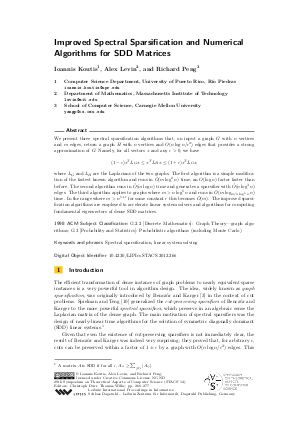Document# Improved Spectral Sparsification and Numerical Algorithms for SDD Matrices

### Authors Ioannis Koutis, Alex Levin, Richard Peng## File

LIPIcs.STACS.2012.266.pdf
• Filesize: 0.62 MB
• 12 pages

## Cite As

Ioannis Koutis, Alex Levin, and Richard Peng. Improved Spectral Sparsification and Numerical Algorithms for SDD Matrices. In 29th International Symposium on Theoretical Aspects of Computer Science (STACS 2012). Leibniz International Proceedings in Informatics (LIPIcs), Volume 14, pp. 266-277, Schloss Dagstuhl - Leibniz-Zentrum für Informatik (2012)
https://doi.org/10.4230/LIPIcs.STACS.2012.266

## Abstract

We present three spectral sparsification algorithms that, on input a graph G with n vertices and m edges, return a graph H with n vertices and O(n log n/epsilon^2) edges that provides a strong approximation of G. Namely, for all vectors x and any epsilon>0, we have (1-epsilon) x^T L_G x <= x^T L_H x <= (1+epsilon) x^T L_G x, where L_G and L_H are the Laplacians of the two graphs. The first algorithm is a simple modification of the fastest known algorithm and runs in tilde{O}(m log^2 n) time, an O(log n) factor faster than before. The second algorithm runs in tilde{O}(m log n) time and generates a sparsifier with tilde{O}(n log^3 n) edges. The third algorithm applies to graphs where m>n log^5 n and runs in tilde{O}(m log_{m/ n log^5 n} n time. In the range where m>n^{1+r} for some constant r this becomes softO(m). The improved sparsification algorithms are employed to accelerate linear system solvers and algorithms for computing fundamental eigenvectors of dense SDD matrices.
##### Keywords
• Spectral sparsification
• linear system solving

## Metrics

• Access Statistics
• Total Accesses (updated on a weekly basis)
0